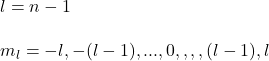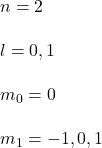## The quantum state of a particle can be specified by giving a complete set of quantum numbers (n,l, ml, ms). How many different quantum state

Question

The quantum state of a particle can be specified by giving a complete set of quantum numbers (n,l, ml, ms). How many different quantum states are possible if the principal quantum number is n = 2? To find the total number of allowed states, first, write down the allowed orbital quantum numbers l, and then write down the number of allowed values of ml for each orbital quantum number. Sum these quantities, and then multiply by 2 to account for the two possible orientations of spin. Express your answer as an integer.

in progress 0
6 months 2021-07-17T08:31:32+00:00 1 Answers 3 views 0

number of quantum states =  8

Explanation:

To find the total number of allowed states you take into account the following relations:in this case you have:furthermore, for each n,l,ml quantum state you have two additional states due to the spin of the electrons.

then, you have (n,l,ml) = (2,0,0), (2,1,-1), (2,1,0), (2,1,1) and with the spin:

number of quantum states = 2*(1+3) = 8